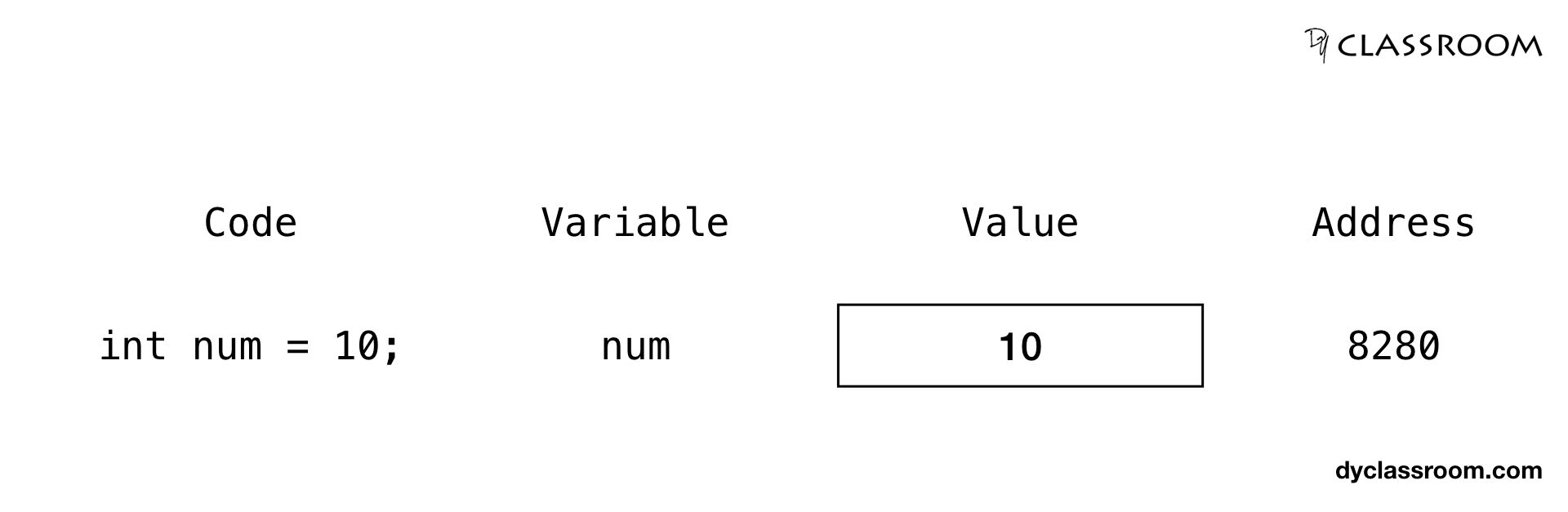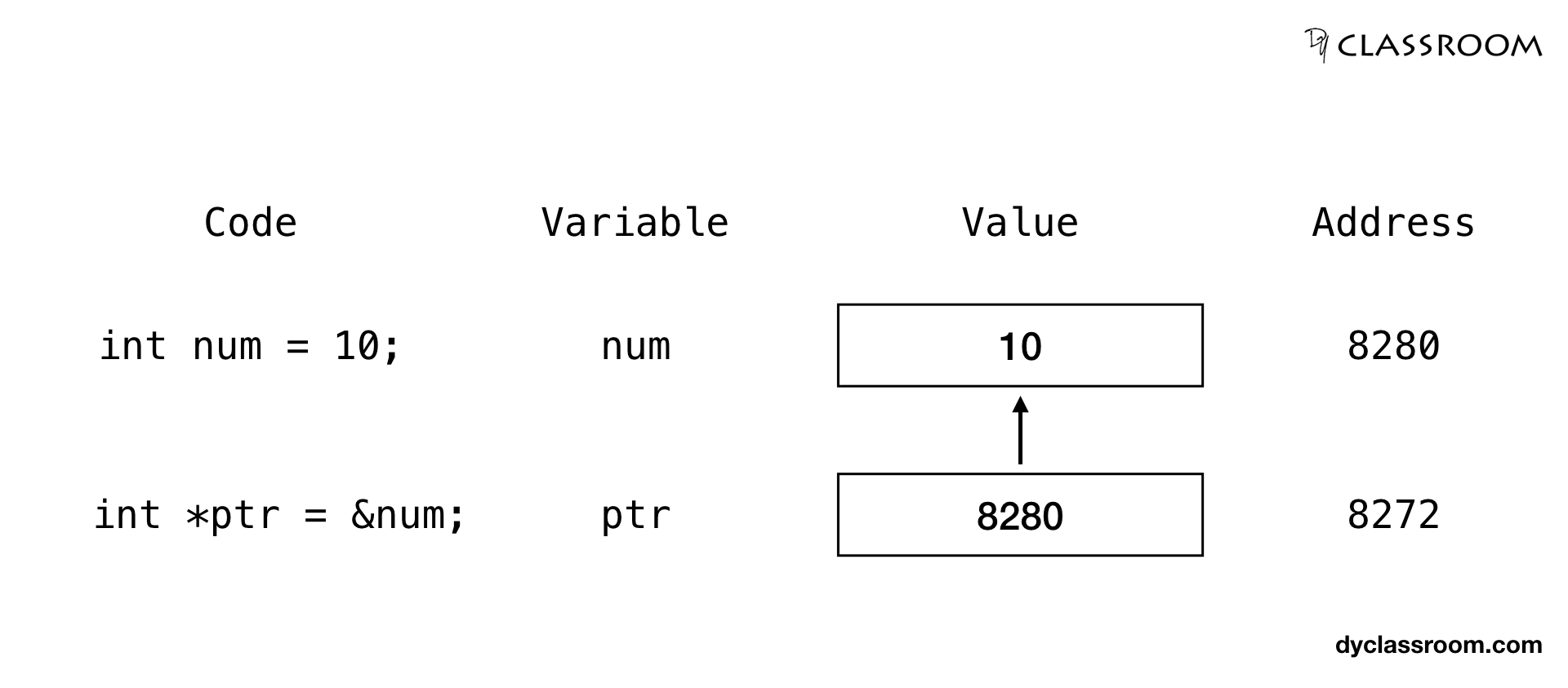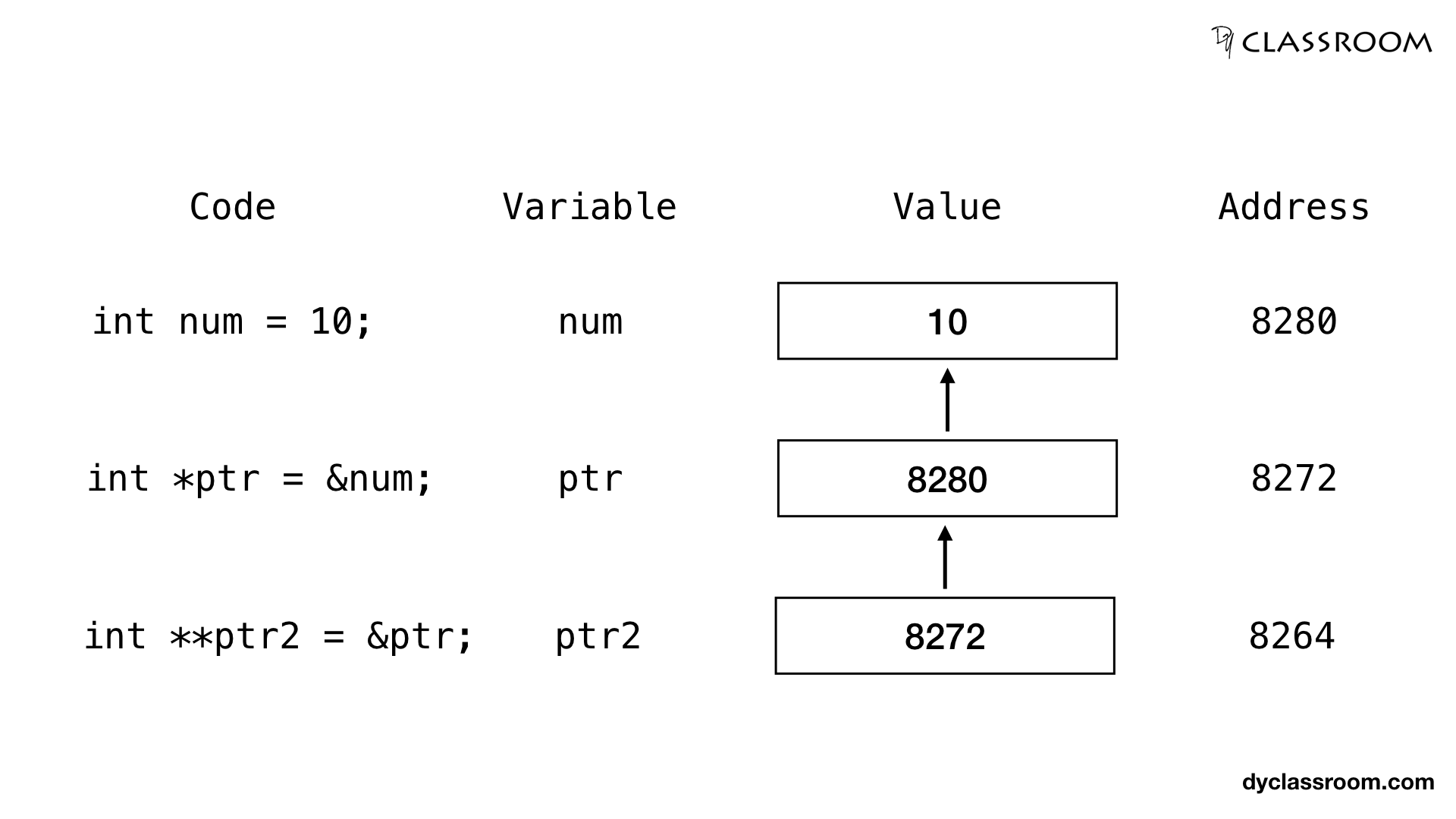# C - Pointers Chaining

C ProgrammingIn this tutorial we will learn to chain pointer variables in C programming language.

In the previous tutorial Pointers and Variables we learned how to manipulate data stored in a variable using pointer variable.

Now we will be chaining some more pointer variables to manipulate other variables.

Note! Complexity increases as we chain more pointers.

## Creating an integer variable

We will start by creating an integer variable `num` and assign value 10 to it.

``````int num = 10;
``````

So, three things happens on writing the above code.

• A memory location is allocated to store the integer variable.
• The value 10 is stored in the memory location.
• The memory location is referred by the variable name `num`.In the above image it is shown that the integer variable `num` gets the memory location 8280 and in that location the value 10 is stored.

## Creating integer pointer variable for the integer variable

Now we will create an integer pointer variable `ptr` that will point at the integer variable `num`.

For this we have to save the address of the `num` variable in the `ptr` variable.

To get the address of a variable we use the address of `&` operator.

``````// integer variable num
int num = 10;

// pointer variable pointing at num
int *ptr = &num;
``````In the above image it is shown that the integer pointer variable `ptr` gets the memory location 8272 and in that location the address of the `num` variable i.e., 8280 is stored. So, ptr variable is pointing at the num variable.

## Creating the second integer pointer variable for the first integer pointer variable

To create a second pointer variable to point at the first pointer variable we use the following syntax.

``````dataType **secondPtr = &firstPtr;
``````

In the following example we are creating the second integer pointer variable `ptr2` that points at the first integer pointer variable `ptr` which is pointing at the integer variable `num`.

``````int **ptr2 = &ptr;
``````In the above image it is shown that the second integer pointer variable `ptr2` gets the memory location 8264 and in that location the address of the first pointer variable `ptr` i.e., 8272 is stored. So, ptr2 variable is pointing at the ptr variable.

## Update value of integer variable via second integer pointer variable

For this we have to first get the address of the variable `num` which is stored in integer pointer variable `ptr`.

We use the value at the address of `*` operator to get the value stored at a given memory address.

To get the address of the integer variable `num` which is stored as a value in integer pointer variable `ptr` we have to write the following.

``````*ptr
``````

And to get the address of the integer pointer variable `ptr` which is stored as a value in integer pointer variable `ptr2` we have to write the following.

``````*(*ptr2)
``````

So, `*ptr2` is pointing at `ptr` and `*ptr` is pointing at `num`.

And `*(*ptr2)` is giving us the value stored at the memory address (in this case 8280) that is allocated to the `num` variable.

So, to update the value stored in the integer variable `num` via the second integer pointer variable `ptr2` we have to write the following.

``````// updating the value of num via ptr2
*(*ptr2) = 20;
``````

### Complete code

``````#include <stdio.h>

int main(void) {

// num variable
int num = 10;

// ptr pointer variable
int *ptr = NULL;

// second ptr2 pointer variable
int **ptr2 = NULL;

// assigning the address of num to ptr
ptr = &num;

// assigning the address of ptr to ptr2
ptr2 = &ptr;

// printing the value of num - Output: 10
printf("num: %d\n", num);
printf("num via ptr: %d\n", *ptr);
printf("num via ptr2: %d\n", *(*ptr2));

// updating the value of num via ptr2
printf("Updating value of num via ptr2...\n");
*(*ptr2) = 20;

// printing the new value of num - Output: 20
printf("num: %d\n", num);
printf("num via ptr: %d\n", *ptr);
printf("num via ptr2: %d\n", *(*ptr2));

return 0;
}
``````

Output:

``````num: 10
num via ptr: 10
num via ptr2: 10
Updating value of num via ptr2...
num: 20
num via ptr: 20
num via ptr2: 20
``````